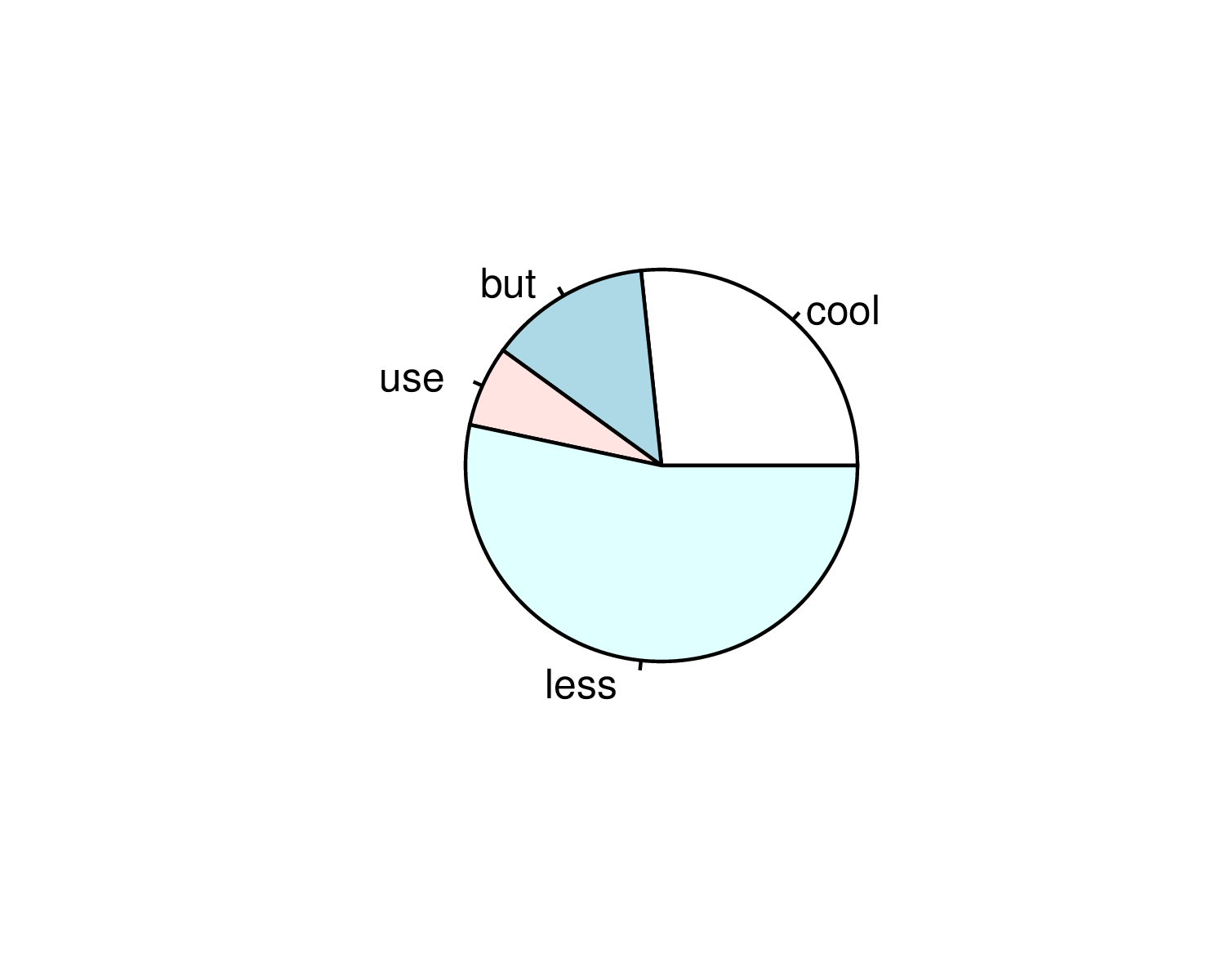# devoutpdf`devoutpdf` is a hand-crafted PDF graphics device written in plain R.

It achieves this by invoking the `devout` package to do all the interfacing between the C++ side and the R side.

Drawing commands which sent to the graphics device are used to construct a `minipdf` document.

### Why would you want this?

Given that `pdf()` and `cairo_pdf()` devices come with R, what’s the point of a third PDF output device?

• Hacking your own graphics device gives a significant amount of control over the actual output
• You can learn how a graphics device works - it’s just R, so there’s no C++ code to sift through.
• Use as a template to write your own graphics device for something bespoke e.g.  pen plotter output or a laser projection driver!
• Glitch the output at the device level e.g. for each line, perhaps draw multiple overlapping lines with randomly jittered endpoints to simulate a ‘pencil sketch’ effect.

### The R code

The R code for this device is a single file about 300 lines long - most of which is whitespace and comments. Check it out on github

### ToDo

• Helvetica font is currently used regardless of what the user specifies. This is a deficiency that first needs to be fixed in `minipdf`.

## Installation

You can install from GitHub with:

``````# install.packages("devtools")
devtools::install_github("coolbutuseless/devout")    # graphics device shim
devtools::install_github("coolbutuseless/minipdf")   # PDF document builder
devtools::install_github("coolbutuseless/devoutpdf") # Devout PDF graphics device``````

## Example: ggplot scatterplot

``````devoutpdf::pdfout(filename = "/img/devoutpdf/test1.pdf", width=5, height=4)
ggplot(mtcars) +
geom_point(aes(mpg, wt)) +
labs(title = "test1") +
theme_bw()
invisible(dev.off())``````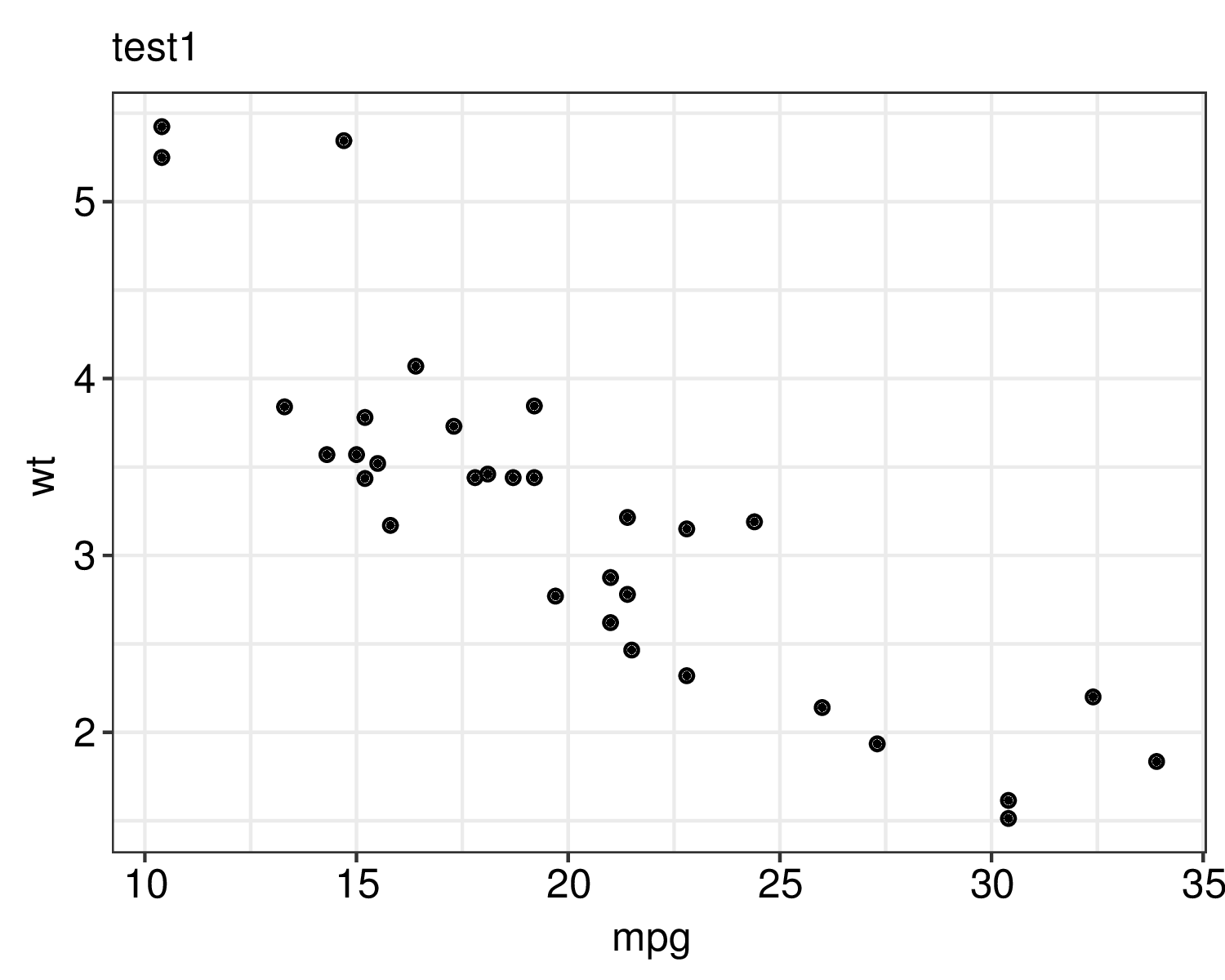## Example: ggplot bar plot

``````devoutpdf::pdfout(filename = "/img/devoutpdf/test2.pdf", width = 6, height = 4)
ggplot(mtcars) +
geom_bar(aes(as.factor(cyl), fill = as.factor(cyl))) +
labs(title = "test2")
invisible(dev.off())``````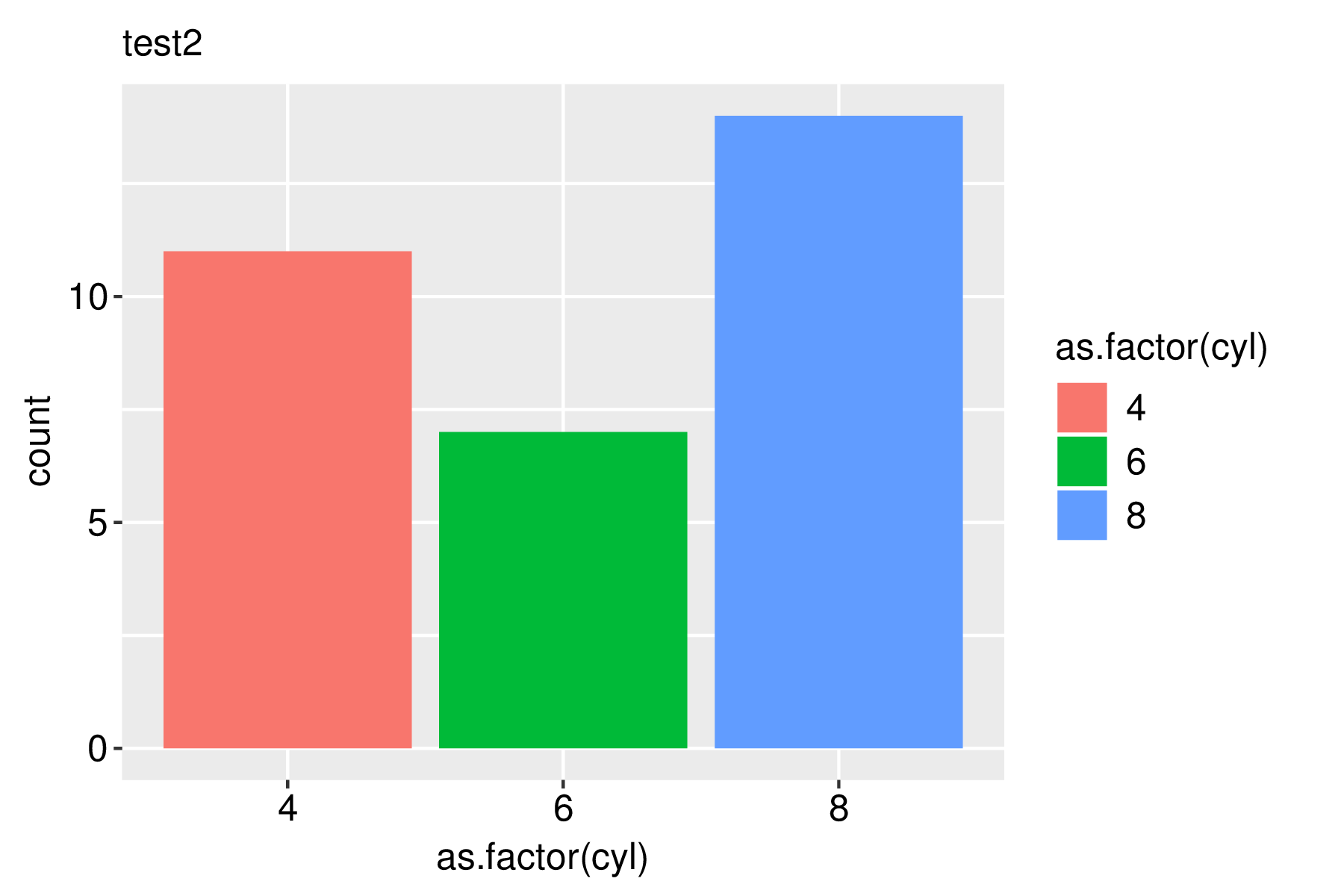## Example: ggplot density plot

``````devoutpdf::pdfout(filename = "/img/devoutpdf/test3.pdf", width = 6, height = 4)
ggplot(mtcars) +
geom_density(aes(mpg, fill = as.factor(cyl)), alpha = 1, size = 2) +
theme_bw()
invisible(dev.off())``````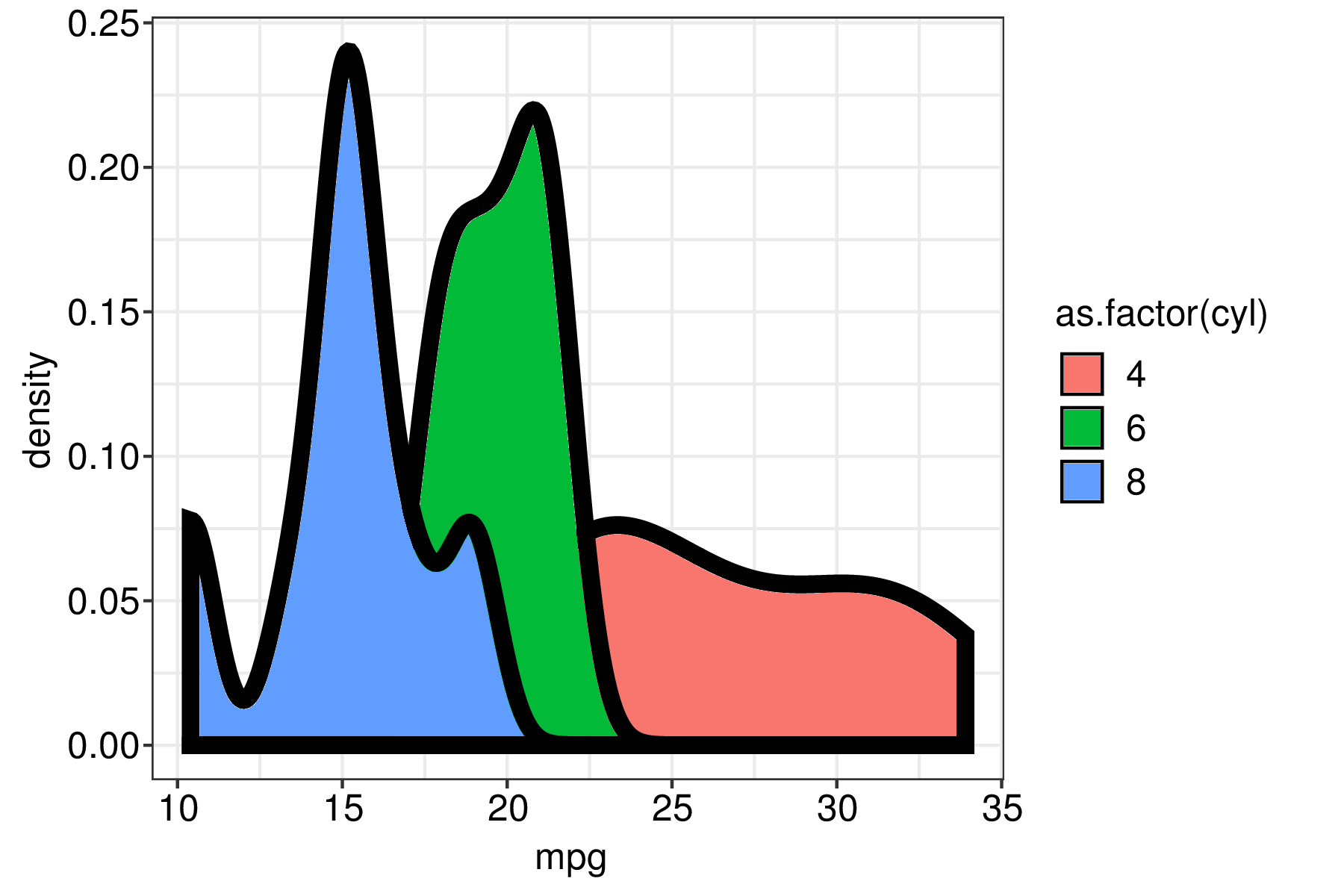## Example: tmap world plot

``````library(tmap)
data("World")

devoutpdf::pdfout(filename = "/img/devoutpdf/test4.pdf", width = 5, height = 4)
tm_shape(World) +
tm_polygons("HPI")
invisible(dev.off())``````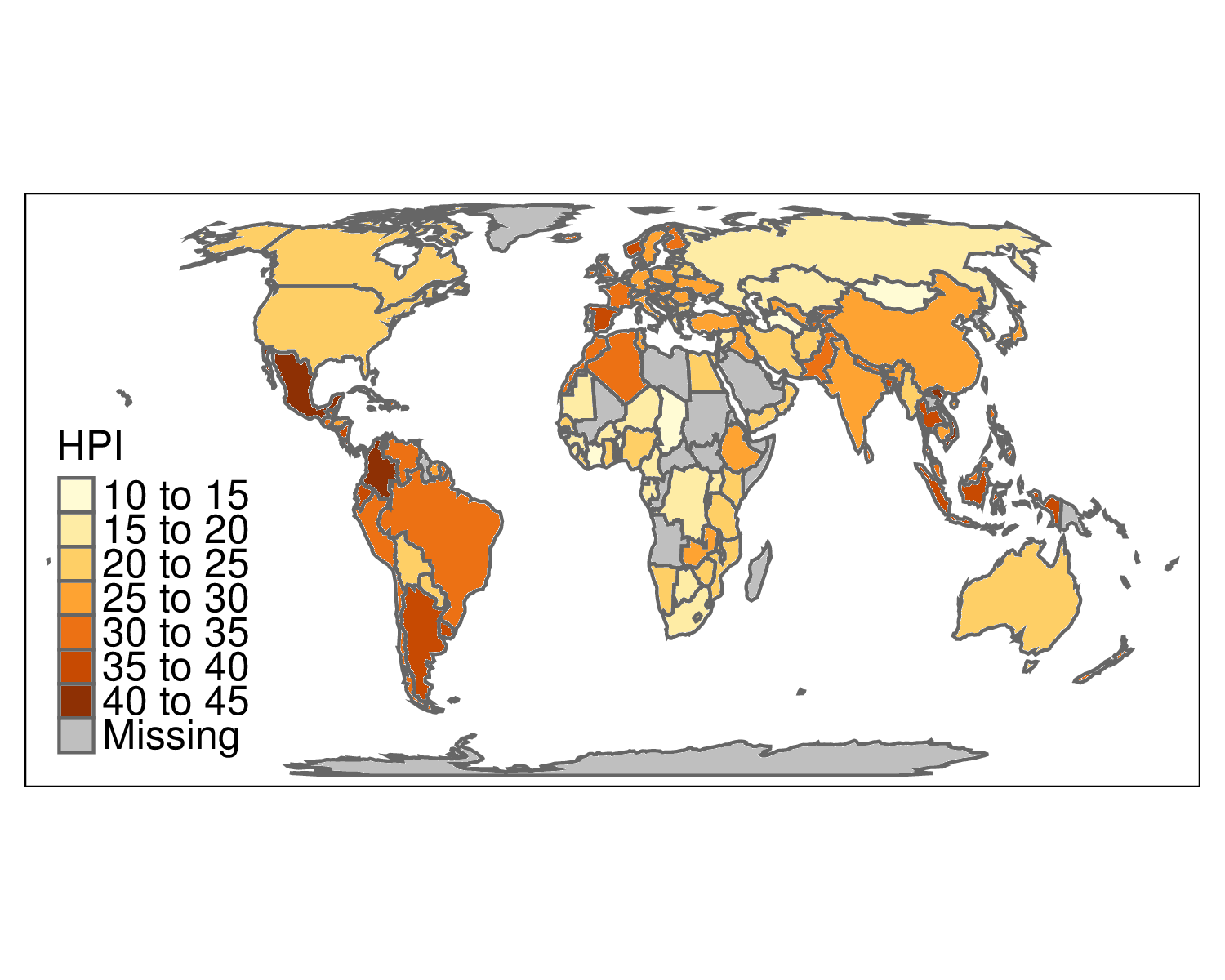## Example: base graphics pie chart

``````devoutpdf::pdfout(filename = "/img/devoutpdf/test5.pdf", width = 5, height = 4)
pie(c(cool = 4, but = 2, use = 1, less = 8))
invisible(dev.off())``````